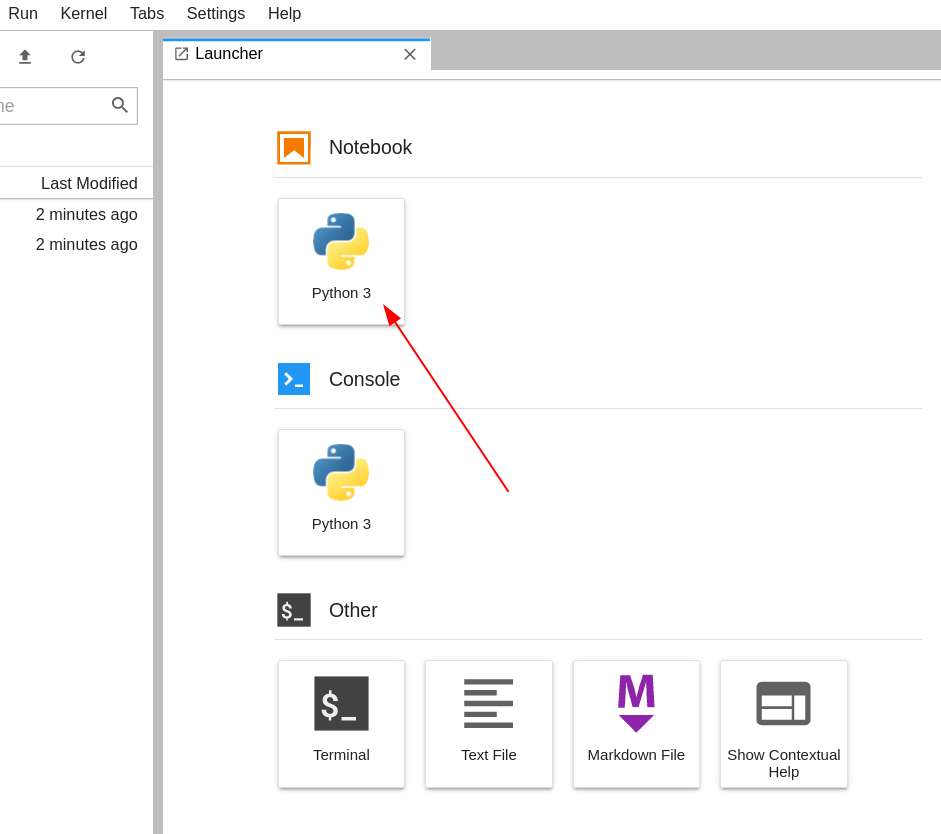# A first computational notebook

Objectives

• Get started with notebooks for analysis.

• Get a feeling for the importance of execution order.

Instructor note

• 10 min teaching

• 10 min exercises

## Creating a computational narrative

Let’s create our first real computational narrative in a Jupyter notebook (adapted from Python and R data analysis course at Aalto Science IT).

Imagine you are on a desert island and wish to compute pi. You have a computer with you with Python installed but no math libraries and no Wikipedia.

Here is one way of doing it - “throwing darts” by generating random points within a square area and checking whether the points fall within the unit circle.

Hint: Opening a webpage inside JupyterLab

If you would like to copy-paste content from this webpage into your Jupyter notebook, a cool way of doing it is to open this page inside an IFrame:

from IPython.display import IFrame
IFrame(src="https://coderefinery.github.io/jupyter/first-notebook/", width='100%', height='500px')


## Launching JupyterLab notebook

In your terminal create a folder or navigate to a folder where you would like the new notebook to appear.

Then launch JupyterLab:

$jupyter-lab  This opens JupyterLab in your browser. Click on the Python 3 tile.JupyterLab opened in the browser. Click on the Python 3 tile. If you prefer to select in which browser to open JupyterLab, use: $ jupyter-lab --no-browser


## An example computational notebook

Exercise/demonstration: Calculating pi using Monte Carlo methods

This can be either done as a 20 minute exercise or as a type-along demo.

Each numbered item will be a new cell. Press SHIFT+ENTER to run a cell and create a new cell below.

1. Create a new notebook, name it, and add a heading (markdown cell).

# Calculating pi using Monte Carlo methods

2. Document the relevant formulas in a new cell (markdown cell):

## Relevant formulas

- square area: $s = (2 r)^2$
- circle area: $c = \pi r^2$
- $c/s = (\pi r^2) / (4 r^2) = \pi / 4$
- $\pi = 4 * c/s$

3. Add an image to explain the concept (markdown cell):

## Image to visualize the concept

![Darts](https://raw.githubusercontent.com/coderefinery/jupyter/main/example/darts.svg)

4. Import two modules that we will need (code cell):

# importing modules that we will need

import random
import matplotlib.pyplot as plt

5. Initialize the number of points (code cell):

# initializing the number of "throws"

num_points = 1000

6. “Throw darts” (code cell):

# here we "throw darts" and count the number of hits

points = []
hits = 0
for _ in range(num_points):
x, y = random.random(), random.random()
if x*x + y*y < 1.0:
hits += 1
points.append((x, y, "red"))
else:
points.append((x, y, "blue"))

7. Plot results (code cell):

# unzip points into 3 lists
x, y, colors = zip(*points)

# define figure dimensions
fig, ax = plt.subplots()
fig.set_size_inches(6.0, 6.0)

# plot results
ax.scatter(x, y, c=colors)

8. Compute the estimate for pi (code cell):

# compute and print the estimate

fraction = hits / num_points
4 * fraction


Here is the notebook: https://github.com/coderefinery/jupyter/blob/main/example/darts.ipynb (static version, later we will learn how to share notebooks which are dynamic and can be modified).

Instructor note

Demonstrate out-of-order execution problems and how to avoid them:

• Add a cell at the end of the notebook which redefines num_points

• Then run the cell which computes the pi estimate

• Then demonstrate “run all cells”

## Notebooks in other languages

(Optional exercise) Installing a kernel for your favorite language

Want to use Jupyter for other programming languages than Python? Head over to this list of Jupyter kernels and find the kernel for your favorite language. Follow the link to installation instructions and try to make it work on your machine!

## Discussion

What do we get from this?

• With code separate from everything else, you might just send one number or a plot to your supervisor/collaborator for checking.

• With a notebook as a narratives, you send everything in a consistent story.

• A reader may still just read the introduction and conclusion, but they can easily see more - and try changes themselves - if they want.